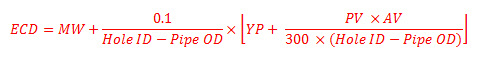# Equivalent Circulating Density (ECD) Using Yield Point for MW More than 13 ppg

Another equation to determine equivalent circulating density is to use yield point.This formula below is used to calculate the ECD and it is good for mud weight more than to 13.0 ppgWhere:

ECD is equivalent circulating density in ppg.

MW is mud weight in ppg.

Hold ID is inside diameter of hole in inch.

Pipe OD is outside diameter of pipe in inch.

YP is mud yield point.

YP can be calculated by these following equations.

YP = Reading at 300 rpm – PV

PV = Reading at 600 rpm – Reading at 300 rpm

AV is annular velocity in ft/min

AV can be determined by the following equation.

AV in ft/min = (24.5 x Q) ÷ (Dh2 – Dp2)

where

Q = flow rate in gpm

Dh = inside diameter of casing or hole size in inch

Dp = outside diameter of drill pipe, drill collars, or tubing in inch

Determine the ECD with the following information

MW = 13.5 ppg

Reading at 300 = 25

Reading at 600 = 40

Hole diameter = 6.2

Pipe diameter = 4

Flow rate = 200 gpm

AV in ft/min = (24.5 x 200) ÷ (6.22 – 42) = 218.4 ft/min

PV = 40 – 25 = 15

YP = 25- 15 = 10ECD = 14.2 ppg

If you have mud weight less than 13 ppg, please read this article ” Equivalent Circulating Density (ECD) Using Yield Point for MW less than 13 ppg

Additional articles about Equivalent Circulating Density (ECD) are as follows:

Equivalent Circulating Density (ECD) in ppg

Equivalent Circulation Density (ECD) with complex engineering equations

Effect of Frictional Pressure on ECD while reverse circulation

Effect of Frictional Pressure on ECD while forward circulation

Share the joyWorking in the oil field and loving to share knowledge.

### 8 Responses to Equivalent Circulating Density (ECD) Using Yield Point for MW More than 13 ppg

1.Mouhanad Habbal says:

May I ask , Do you use formulas in the field ??

•DrillingFormulas.Com says:

Yes.

2.tulio says:

I think this formula can’t be used in the field because you may have several pipe sections like DC, HW, 5″ DP , 4″ DP , 3.5″ DP and so on , so the ECD will be different around every pipe section.
I think the best formula for ECD to use is that one based on annular pressure loss.

•DrillingFormulas.Com says:

This is one of the empirical equations used to calculate ECD. It just give you rough figure which might not be accurate as real measurement (PWD).

3.kresimer zubac says:

I think this formula is not accurate

•DrillingFormulas.Com says:

This formula is just for rough estimation so you will get an ideal how much ECD is.

4.khalil says:

This formula does not include pipe length and well depth.

•DrillingFormulas.Com says:

This is a quick calculation that you can use a simple calculator to estimate the ECD.

This site uses Akismet to reduce spam. Learn how your comment data is processed.